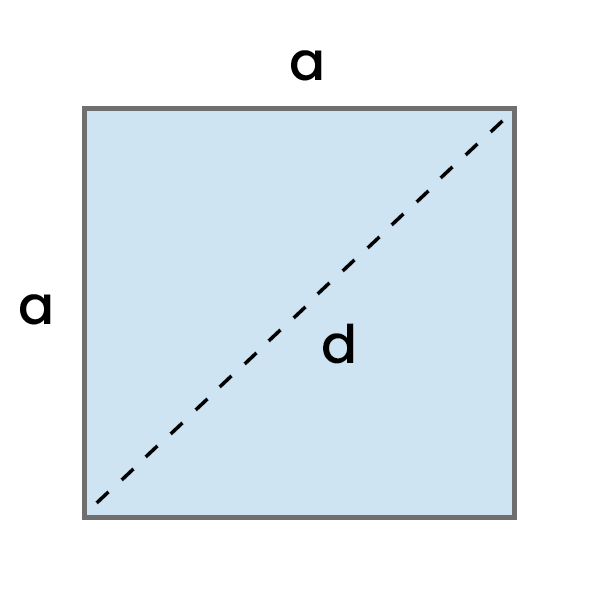# Area of a Square Calculator

Calculate the area of a square based on the entered length of one side or diagonal in your chosen units, and see the detailed step-by-step calculation process.Result:

## How to use this calculator?

1. Enter the Side length (a) or Diagonal length (d) in the chosen units.
2. See the calculated Area of a Square. If you want to, you can change its unit.
3. See the detailed step-by-step Calculation process.

## How to calculate Area of a Square?

Calculating the area of a square is easy, but the method depends on the data you have.

The simplest way to calculate the area of a square is by knowing the length of its side, but you can also calculate it based on the length of the diagonal.

### With Side Length

Multiply the length of one side by itself.

Area of a Square = 2a = a * a
• a - Side Length
Example: Calculate the area of a square with a side length of 5 cm.

Area = a * a

Area = 5 cm * 5 cm

Area = 25 cm²

### With Diagonal

Multiply the diagonal length by itself to get d2, and then divide the result by 2.

Area of a Square = d2 / 2
• d - Diagonal Length
Example: Calculate the area of a square with a diagonal length of 12 cm.

Area = d² / 2

Area = 12 cm * 12 cm / 2

Area = 144 cm² / 2

Area = 72 cm²